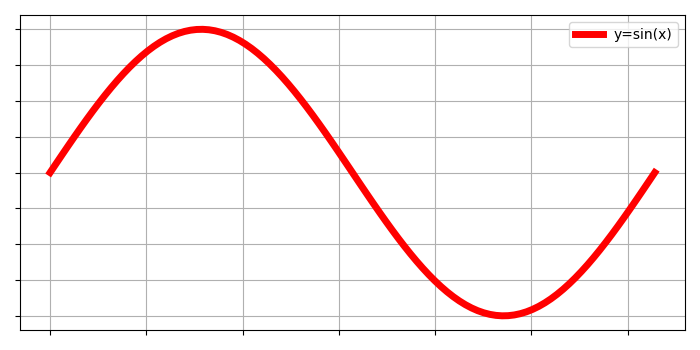# Remove the X-axis ticks while keeping the grids (Matplotlib)

To remove the X-ticks while keeping the grids, we can take the following steps−

• Use gca() method to get the current axes, creating one if necessary.
• Plot the x and np.sin(x) using plot() method with linewidth=5, label y=sin(x).
• Remove yticks and xticks by passing empty array in the argument of set_xticklabels and set_yticklabels methods respectively.
• Configure grid lines by putting flag as True.
• Place the legend for the plot label in the argument.
• To display the figure, use show() method.

## Example

import matplotlib.pyplot as plt
import numpy as np
plt.rcParams["figure.figsize"] = [7.00, 3.50]
plt.rcParams["figure.autolayout"] = True
x = np.linspace(0, 2*np.pi, 100)
ax = plt.gca()
ax.plot(x, np.sin(x), c='r', lw=5, label='y=sin(x)')
ax.set_xticklabels([])
ax.set_yticklabels([])
ax.grid(True)
plt.legend(loc="upper right")
plt.show()

## Output